# What is DataWeave? Part 3: Variables, Flow Control, and Functions

We would like to thank MuleSoft Ambassador Joshua Erney for their contribution to this developer tutorial.

In the previous tutorial, we learned how to use some of the most popular selectors to read data in DataWeave. This tutorial will cover variables, Boolean operators, flow control, and functions. By the end of this tutorial you will understand:

• How to create and use variables
• Which are the Boolean operators
• How to use `if/else` expressions
• The basics of pattern matching
• How to create and use functions

If this is your first time learning about DataWeave, we encourage you to check out this other tutorial to learn about the online DataWeave Playground. With this tool, you will be able to try out the scripts you will see in this tutorial right from your browser.

## Variables

Like other languages, DataWeave has variables so that you can store values to use later on in your script. Think of variables as a container for your data. The name you give to the variable is just like putting a label on the outside of the container so you can easily find it later. Variables are useful for assigning names to values and storing a calculation that would otherwise need to be repeated. To set a variable, use the following syntax:

An expression is something that returns a value or is a value itself. Here’s an example of setting a variable to an explicit value:

You can also use variables to store the results of calculations for later use.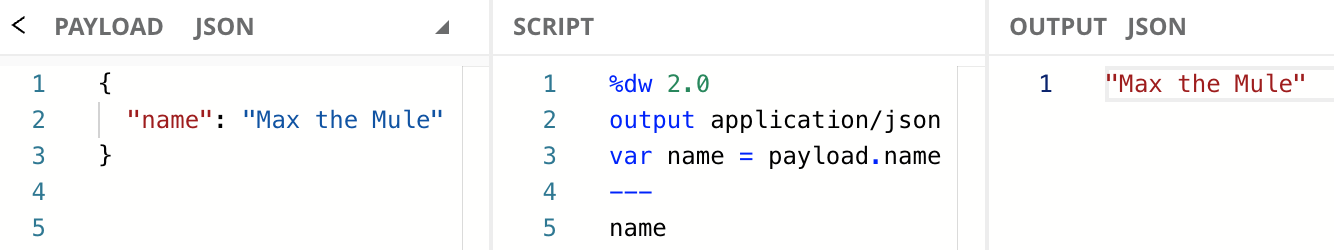## Boolean Operators

Flow control is used when you want to execute certain parts of your code in some situations, while not executing others. In other words, it’s a way to add logic to your scripts.

A common use case for variables is to store the result of some kind of Boolean operation. Think of a Boolean operation as an expression that returns some value if some criteria is met, and returns another value if the criteria is not met. The simplest Boolean operations use equality, relational, and logical operators. You might be familiar with these from other languages:

 A `>` B Greater than A `<` B Less than A `>=` B Greater than or equal to A `<=` B Less than or equal to A `==` B Equal to A `~=` B Similar to* `!`A Logical negation** `not` A Logical negation** A `and` B Logical and A `or` B Logical or

*Tries to coerce two values of different types to the same type and compare them (e.g., `"1" ~= 1` would evaluate to `true`)

**Both of these operators perform logical negation (i.e. both `!true` and `not true` evaluate to `false`). However, `!` has very high precedence; it always operates first in a chain of logical operators. `not`, on the other hand, has low precedence. It typically operates last in a chain of logical operators. For example, `!true or true` evaluates to `true`, whereas `not true or true` evaluates to `false` because `true or true` is evaluated first to be `true`, then negated by `not` to be `false`.

## Flow Control

### if else

For example, you might want to check if a number is greater than 100 so that you can inform a system whether or not to buy something. To do this, we will use the if/else expression, which is formatted like this:

There are cases in DW where parentheses are optional, but it’s important to note the criteria must be surrounded by parentheses in if/else expressions.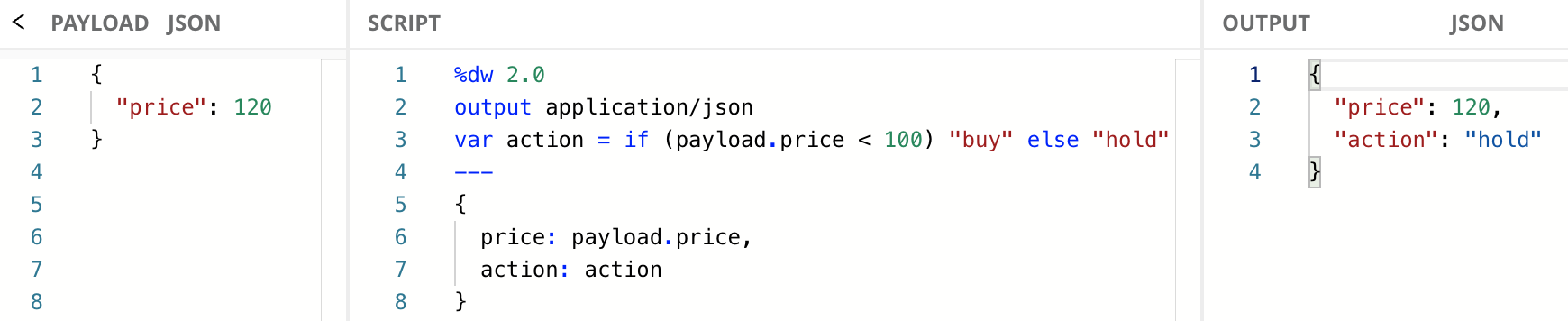If you’re familiar with popular languages like Java, or C#, you’ll notice the way DataWeave implements if/else is much closer to a ternary expression than the if/else statements you see in those languages. The difference is very simple. DW uses if/else expressions that return values. Java and C# use if/else statements that do not return values.

### else if

if/else expressions are chainable, meaning you can do multiple checks before you return any data. Here’s the syntax for how that works:

You can have as many of these if/else chains as necessary. Imagine you had a third option in addition to `"buy"` and `"hold"`: `"sell"`. You could chain if/else expressions together to account for these additional criteria.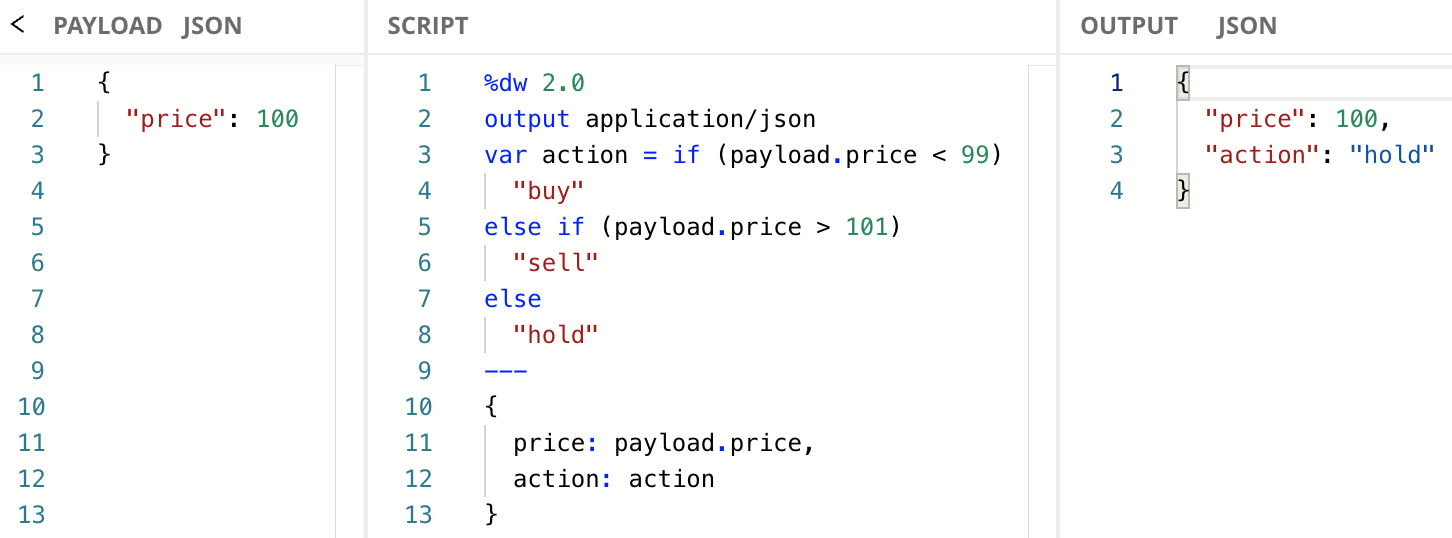### Intro to Pattern Matching

Pattern matching is another method of flow control, but it does quite a bit more under the hood than the if/else expression does, and the syntax is a little more complicated. Like the if/else expression, pattern matching also returns a single value. Here’s a simplification of how pattern matching expressions are formatted:

The easiest way to understand basic pattern matching is to show an example.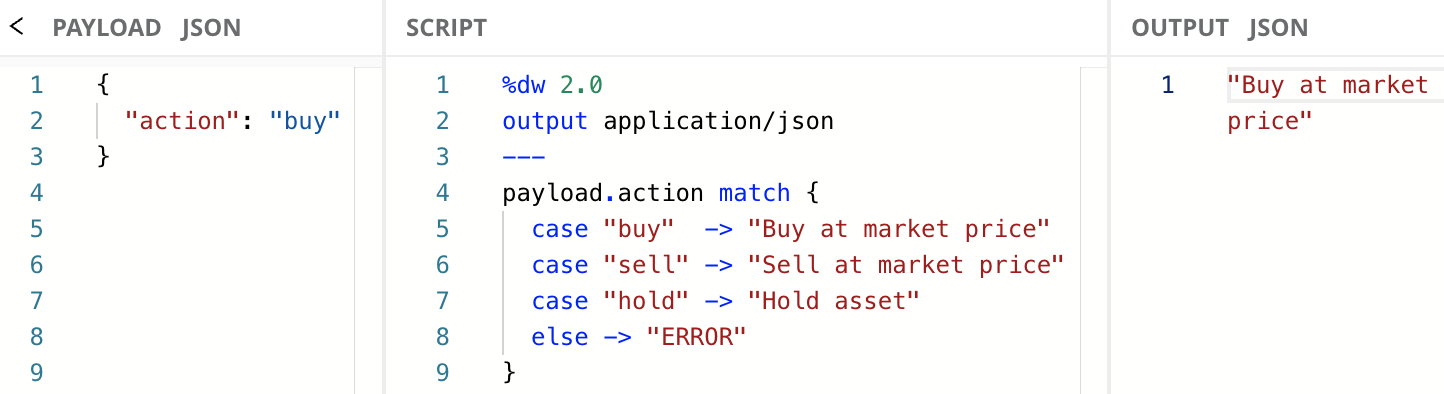## Functions

Functions are one of DataWeave’s most important tools. They allow us to conveniently reuse functionality and create functionality on the fly when reuse isn’t necessary. We create functions in the declarations section of the script using the `fun` keyword. This associates a set of functionality with a name. Here’s the basic syntax:

It’s a good practice to put the body on a new line and indent, like this:

Here’s a simple example of creating a function and calling it: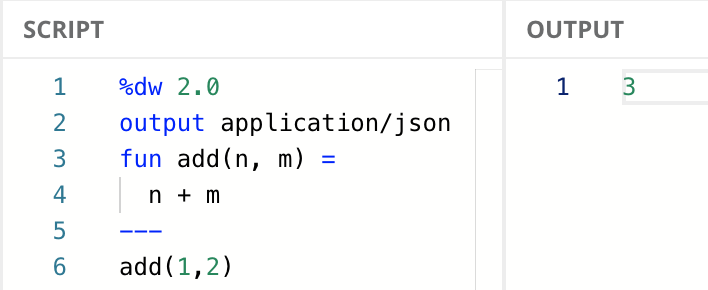Notice that there is no return keyword. A return keyword isn’t needed because almost everything in DataWeave is an expression, and all expressions return data.

This way of calling functions is called prefix notation. You’re likely familiar with prefix notation from languages like Java, JavaScript, and Python. With prefix notation, the function name comes before the arguments. DataWeave supports another notation: infix notation.

### Infix notation

In DataWeave, if a function takes two arguments, like the previous `add` function, you can call it with infix notation. Infix notation has the following syntax:

Here’s how the code above would look if we called `add` using infix notation: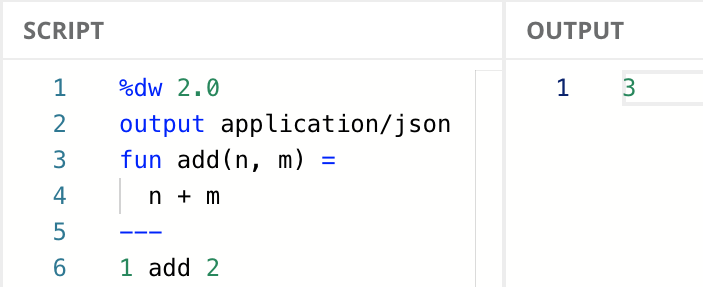## Next Steps

In this tutorial, we learned about variables, Boolean operators, flow control, and functions. We now know:

Now that we learned about named functions in DataWeave, in the next tutorial we’ll learn about a different kind of function called lambda, about the dollar-sign syntax, and we’ll see more examples using the infix notation.

Click on the Next button below to continue to the next tutorial.